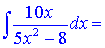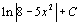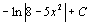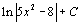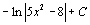Math Calculators, Lessons and Formulas

It is time to solve your math problem

mathportal.org
Integration Techniques: (lesson 1 of 4)

## Integration by Substitution

The substitution method turns an unfamiliar integral into one we can evaluate. In other words, substitution gives us a simpler integral involving the variable u. This lesson shows how the substitution technique works.

Let's now review the five steps for integration by substitution.

Step 1: Choose a new variable u

Step 2: Determine the value dx

Step 3: Make the substitution

Step 4: Integrate resulting integral

Step 5: Return to the initial variable x

### Integration by substituting u = ax + b

These are typical examples where we use the method of subsitution.

Example 1: Evaluate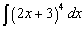Solution:

Step 1: Chose a substitution function u

The substitution function is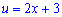Step 2: Determine the value dxStep 3: Make the substitution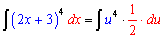Step 4: Integrate resulting integral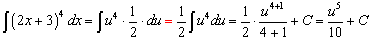Step 5: Return to the initial variable: x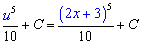So, the solution is: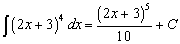Example 2: Evaluate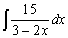Solution:

Step 1: Chose a substitution function u

The substitution function is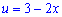Step 2: Determine the value dx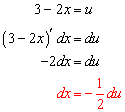Step 3: Make the substitution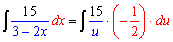Step 4: Integrate resulting integral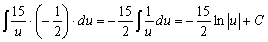Step 5: Return to the initial variable: x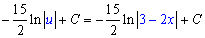The solution is: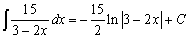Exercise 1: Evaluate using substitution u = ax + b

Level 1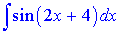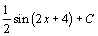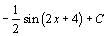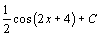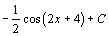Level 2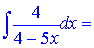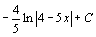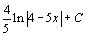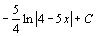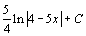### More complicated examples

The steps for doing integration by substitution in this section are the same as the steps for previosu one, but we have to chose our substitution function wisely.

Example 3: Find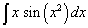Solution: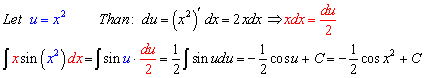Example 4: Find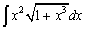Solution: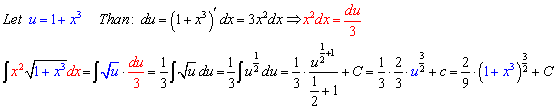Example 5: Find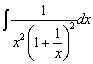Solution: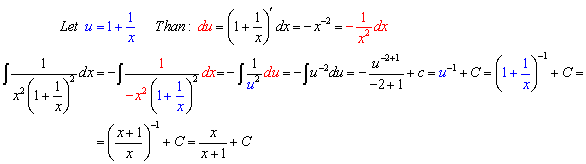Exercise 2: Evaluate using substitution

Level 1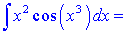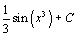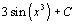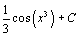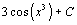Level 2